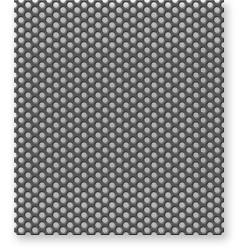# Resonant Sound Absorbers (Part III)

### The Absorption Bandwidth

Not only are we interested in the maximum value of sound absorption that occurs at the resonance frequency of a tuned absorber, but we want to know whether the resonance peak is broad or narrow. In fact, in designing a tuned resonant sound absorber, we want to achieve the required bandwidth.

As a practical matter, we can characterize the absorption bandwidth of a resonant sound absorber by determining the two frequencies, f2 and f1 (above and below the resonance frequency, respectively) at which the absorption has dropped to half its value at resonance. For frequencies below f1 and above f2, the absorption of the tuned absorber is relatively insignificant.

The difference between f2 and f1 is called the "Half-Power Bandwidth" because at all frequencies within this band the sound absorption exceeds half the (maximum) value at resonance:

! 6fH = f2 -f1;

= 27f[1 + (R/pc)] (h/c) fR2;

and

f1,2 = fR :!: (6fH/2)

These quantities are shown in Fig. 25. a

Figure 25. Bell curve of absorption defining Umax, f" f2 and L:.fHo

~ 82

~f/fR f1,2 = fR :!:~ 2

Once the resonance frequency fR is chosen, the breadth of the absorption curve depends only on (1) the depth of the airspace behind the perforated metal and (2) the flow resistance of the filling material.

Assuming that the latter is chosen so as to maximize the maximum absorption at resonance, or to suit some other criterion, then the airspace depth alone governs the resonance bandwidth. If 6fH is small, the absorption band is narrow, and we target a very limited range of frequencies. To absorb a wider band of frequencies effectively requires greater depth for the airspace.

### Combined Effects of Flow Resistance, Filling, and Absorber Dimensions

Figure 26 will help us understand the respective roles of the filling material and the absorber dimensions in determining how the absorber behaves. % R/pc = 1 [3 = 2

Relative Frequency

Figure 26. Frequency dependence of the absorption coefficient of perforated sheet in front of an air cushion filled with absorptive material: various values for R/pc and 13 (see text).

The lower part of the figure shows frequency plots of the sound absorption coefficient for several tuned resonant absorbers, all of which are filled with an absorptive material having R/pc = 1, but with different values for the construction parameter ~.

For the frequency, we use the ratio, f/fR, of actual frequency to the resonance frequency, on a logarithmic scale. On such a scale, the absorption plots show up as bell-shaped curves, symmetrical about the resonance frequency f/fR = 1.

The parameter ~, here, is related to the depth of the absorber and to the "half power bandwidth" 6fH (introduced above) by the following two equations:

~ = c/21TfRh;

The greater the depth of the airspace h, or the higher the resonance frequency fR, the smaller the value of ~.

6fH = (f~~) [1 + (R/pc)].

The smaller the value of ~, or the higher the resonance frequency, or the greater the flow resistance of the filling, the wider the frequency band over which high sound absorption will occur .

In the lower part of Figure 26, the choice of R/pc = 1 causes the sound absorption at resonance (f/fR = 1) to be 100% in all cases. But smaller values for ~ lead to absorption curves that are broader; and high values for ~ lead to narrower curves.

In the upper part of the figure, the value of ~ i~ 2 for all the curves, but different values for R/pc are chosen. Again, for R/pc = 1 the absorption at resonance is 100% .But for R/pc = q.5 and 2.0 the absorption at resonance is nearly as great (amax = 0.89). Also, note that values of R/pc greater than unity lead to broader absorption curves, while values less than unity give narrower curves.